Custom SearchPLACING THE DECIMAL POINT Various methods have been advanced regarding the placement of the decimal point in numbers derived from slide rule computations. Probably the most universal and most easily remembered method is that of approximation. The method of approximation means simply the rounding off of numbers and the mechanical shifting of decimal points in the numbers of the problem so that the approximate size of the solution and the exact position of the decimal point will be seen from inspection. The slide rule may then be used to derive the correct sequence of significant digits. The method may best be demonstrated by a few examples. Remember, shifting the decimal point in a number one place to the left is the same as dividing by 10. Shifting it one place to the right is the same as multiplying by 10. Every shift must be compensated for in order for the solution to be correct. EXAMPLE: 0.573 x 1.45 SOLUTION: No shifting of decimals is necessary here. We see that approximately 0.6 is to be multiplied by approximately 1 1/2. Immediately, we see that the solution is in the neighborhood of 0.9. By slide rule we find that the significant digit sequence of the product is 832. From our approximation we know that the decimal point is to the immediate left of the first significant digit, 8. Thus, 0.573 x 1.45 = 0.832 EXAMPLE: 239 x 52.3 SOLUTION: For ease in multiplying, we shift the decimal point in 52.3 one place to the left, making it 5.23. To compensate, the decimal point is shifted to the right one place in the other factor. The new position of the decimal point is indicated by the presence of the caret symbol. 239.0^ x 5^2.3 Our problem is approximately the same as 2,400 x 5 = 12,000 By slide rule the digit sequence is 125. Thus, 239 x 52.3 = 12,500 EXAMPLE: 0.000134 x 0.092 SOLUTION: Shifting decimal points, we have 0^00.000134 x 0.09^2 Approximation: 9 x 0.0000013 = 0.0000117. By slide rule the digit sequence is 123. From approximation the decimal point is located as follows:         0.0000123 Thus,         0.000134 x 0.092 = 0.0000123 Example: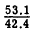SOLUTION: The decimal points are shifted so that the divisor becomes a number between 1 and 10. The method employed is cancellation. Shifting decimal points, we have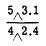Approximation:  5/4 = 1.2 Digit sequence by slide rule:     1255 Placing the decimal point from the approximation:     1.255 Thus,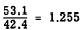ExampleSOLUTION: Shifting decimal points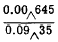Approximation: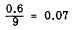Digit sequence by slide rule: 690 Placing the decimal point from the approximation:             0.0690 Thus,Practice problems. Solve the following problems with the slide rule and use the method of approximation to determine the position of the decimal point:Answers: 1. 0.000745 2. 42.4 3. 0.0438 4. 0.212